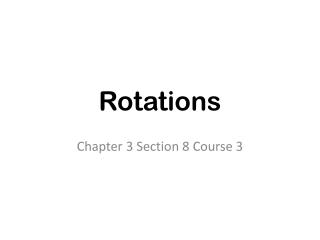# Rotations - PowerPoint PPT PresentationDownload PresentationRotations

RotationsDownload Presentation## Rotations

- - - - - - - - - - - - - - - - - - - - - - - - - - - E N D - - - - - - - - - - - - - - - - - - - - - - - - - - -
##### Presentation Transcript

1. Rotations Chapter 3 Section 8 Course 3

2. Transformations example: earth rotates on its axis

3. Rotation about a point π A rotation – is a turn • The number of degrees an image is rotated is called the angle of rotation. written as rx°(P) = P’ π

4. Rotation in the Coordinate Plane When a figure is rotated 90°, 180° or 270° you can use the following rules. r90°(x,y) =(-y, x) r180°(x,y) =(-x, -y) r270°(x,y) =(y, -x)

5. Example P(-2, 3) 1. r90°(P) = 2. r180°(P) = 3. r270°(P) =

6. Example A(1, -2) 1. r90°(A) = 2. r180°(A) = 3. r270°(A) =

7. Example B(4, 1) 1. r90°(A) = 2. r180°(A) = 3. r270°(A) =

8. Example ABC has vertices A(1, 1), B(1, 6) and C(4, 1). r180°(ABC)

9. Example LMN has vertices L(0, 0), M(3, -5) and N(-2, -2). r90°(LMN)

10. Example L(-2, 5) 1. r90°(L) = 2. r180°(L) = 3. r270°(L) =

11. Example M(-1, -3) 1. r90°(M) = 2. r180°(M) = 3. r270°(M) =

12. Example ABC has vertices A(-2, 1), B(-2, -2) and C(0, 0). r270°(ABC)

13. Rotational Symmetry A figure has rotational symmetry if you can rotated (turn) 180° or less and it exactly matches up with the original figure. Example:

14. Does the figure have rotational symmetry, reflectional symmetry or both. 1. 2. 3. 4.